# Explicit form of interpolating cubic spline and data smoothing

#### 2023-01-08

The package is the result of research, the findings of which will soon be published in an article with an almost identical title Explicit forms of interpolating cubic splines and data smoothing.

## Package Info:

The main purpose of the ICSsmoothing package is to provide functions to support the computation of a clamped interpolating cubic spline ($$\textit{CICS}$$) $$\mathbf{S}$$ of class $$C^2$$ according to its new $$\boldsymbol{explicit}$$ interpolating form $\mathbf{S}=\mathbf{B}\cdot\boldsymbol{\gamma},$ where

$$\hspace{5mm}\mathbf{S}$$ is the vector of $$k$$ spline components,

$$\hspace{5mm}\mathbf{B}$$ is the $$k\times (k+3)$$ matrix of bazis functions and

$$\hspace{5mm}\boldsymbol{\gamma}$$ is the vector of $$k+3$$ spline coefficients.

Thanks to functions $$\textit{cics_unif_explicit}$$ and $$\textit{cics_explicit}$$ one can construct either uniform (nodes are equidistant) or non-uniform (nodes are arbitrary) splines in the explicit interpolating form. Due to further two functions $$\textit{cics_unif_explicit_smooth}$$ and $$\textit{cics_explicit_smooth},$$ one can smooth scattered data points according to regression models based on the explicit interpolating form. Their benefit is the simply and naturally interpretable clamped spline coefficient $$\boldsymbol{\gamma}$$, which contains $$k+1$$ $$y$$-values on the spline curve and its two derivatives at the edges.

rm(list = ls())
library(ICSsmoothing)
## Warning: package 'ggplot2' was built under R version 4.2.2

# Examples of essential functions:

• $$\mathbf{cics\_unif\_explicit(umin, umax, yy, d, ...)}$$

Let $$P=\{[-1,2],[0,3],[1,-2],[2,6]\}$$, i.e. there be given equidistant nodes $$(-1,0,1,2)^{\mathsf{T}}$$ and values $$(2,3,-2,6)^{\mathsf{T}}$$. Let the derivatives in the first and the last node be $$1$$ and $$4$$. The codelines

yy <- c(2, 3, -2, 6)
d <- c(1, 4)
clrs <- c("blue", "red")
expl_spline <- cics_unif_explicit(-1, 2, yy, d, clrs)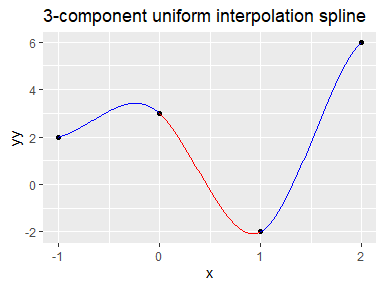provide not only the plot of a uniform $$\textit{CICS}$$ $$\mathbf{S}$$ with 3 components, but from the list $$expl\_spline$$ one can get as well as the building elements of the explicit form $\mathbf{S}=\mathbf{B}\cdot\boldsymbol{\gamma}.$ One can obtain the spline coefficients and the corresponding polynomial expressions of the cubic spline segments

expl_spline$spline_coeffs ## [,1] [,2] [,3] [,4] ## [1,] 3.0 -3.8 -9.6 -4.8 ## [2,] 3.0 -3.8 -9.6 8.4 ## [3,] 21.2 -58.4 45.0 -9.8 expl_spline$spline_polynomials
## List of polynomials:
## []
## 3 - 3.8*x - 9.6*x^2 - 4.8*x^3
##
## []
## 3 - 3.8*x - 9.6*x^2 + 8.4*x^3
##
## []
## 21.2 - 58.4*x + 45*x^2 - 9.8*x^3

or the array $$B_{k\times(k+3)\times 4}$$ and the vector $$\boldsymbol{\gamma_{(k+3)\times 1}}$$

expl_spline$B ## , , 1 ## ## [,1] [,2] [,3] [,4] [,5] [,6] ## [1,] 0.0 1.0 0.0 0.0 0.0000000 0.0000000 ## [2,] 0.0 1.0 0.0 0.0 0.0000000 0.0000000 ## [3,] -0.8 3.2 -3.2 1.8 -0.2666667 -0.9333333 ## ## , , 2 ## ## [,1] [,2] [,3] [,4] [,5] [,6] ## [1,] -0.8 0.2 0.8 -0.2 -0.2666667 0.06666667 ## [2,] -0.8 0.2 0.8 -0.2 -0.2666667 0.06666667 ## [3,] 1.6 -6.4 10.4 -5.6 0.5333333 2.86666667 ## ## , , 3 ## ## [,1] [,2] [,3] [,4] [,5] [,6] ## [1,] 1.4 -2.6 1.6 -0.4 0.4666667 0.1333333 ## [2,] 1.4 -2.6 1.6 -0.4 0.4666667 0.1333333 ## [3,] -1.0 4.0 -8.0 5.0 -0.3333333 -2.6666667 ## ## , , 4 ## ## [,1] [,2] [,3] [,4] [,5] [,6] ## [1,] 1.2 -1.8 0.8 -0.2 0.73333333 0.06666667 ## [2,] -0.6 1.4 -1.4 0.6 -0.20000000 -0.20000000 ## [3,] 0.2 -0.8 1.8 -1.2 0.06666667 0.73333333 expl_spline$gamma
##   2  3 -2  6  1  4

Because of the numerical and not symbolic computation there is a distinction between the matrix $$\mathbf{B}$$ and array $$B$$. The next two examples highlight the role of $$B$$. The codelines

B <- expl_spline$B g <- expl_spline$gamma
i <- 2
k <- 3
B[,,i][k,] %*% g;
##       [,1]
## [1,] -58.4

return the coefficient of $$x^{i-1}$$ in the $$k$$-th spline segment. The auxiliary function $$explicit\_spline$$

explicit_spline(expl_spline$B, expl_spline$gamma) 
##      [,1]  [,2] [,3] [,4]
## [1,]  3.0  -3.8 -9.6 -4.8
## [2,]  3.0  -3.8 -9.6  8.4
## [3,] 21.2 -58.4 45.0 -9.8

implements the computation of the numeric multiplication corresponding to the symbolic matrix multiplication $$\mathbf{B}\cdot\boldsymbol{\gamma}$$, i.e.  \begin{align*} \mathbf{S} =\begin{pmatrix} 3 - 3.8x - 9.6x^2 - 4.8x^3\\ 3 - 3.8x - 9.6x^2 + 8.4x^3\\ 21.2 - 58.4x + 45x^2 - 9.8x^3 \end{pmatrix} =\mathbf{B}\cdot \boldsymbol{\gamma} , \end{align*} where the symbolic matrix $$\mathbf{B}$$ of basis functions and the numeric vector $$\boldsymbol{\gamma}$$ of intepolation spline coefficients, function values and derivatives at the two exterior-nodes, are

\begin{align*} \mathbf{B}^{\mathsf{T}}&=\left(\begin{smallmatrix} -0.8x+1.4x^2+1.2x^3 & -0.8x+1.4x^2-0.6x^3 & -0.8+1.6x-x^2+0.2x^3\\ 1+0.2x-2.6x^2-1.8x^3 & 1+0.2x-2.6x^2+1.4x^3 & 3.2-6.4x+4x^2-0.8x^3\\ 0.8x+1.6x^2+0.8x^3 & 0.8x+1.6x^2-1.4x^3 & -3.2+10.4x-8x^2+1.8x^3\\ -0.2x-0.4x^2-0.2x^3 & -0.2x-0.4x^2+0.6x^3 & 1.8-5.6x+5x^2-1.2x^3\\ -0.2\overline{6}x+0.4\overline{6}x^2+0.7\overline{3}x^3 & -0.2\overline{6}x+0.4\overline{6}x^2-0.2x^3 & -0.2\overline{6}+0.5\overline{3}x-0.\overline{3}x^2+0.0\overline{6}x^3\\ 0.0\overline{6}x+0.1\overline{3}x^2+0.0\overline{6}x^3 & 0.0\overline{6}x+0.1\overline{3}x^2-0.2x^3 & -0.9\overline{3}+2.8\overline{6}x-2.\overline{6}x^2+0.7\overline{3}x^3 \end{smallmatrix}\right),\\ \boldsymbol{\gamma}&=(2,3,-2,6,1,4)^{\mathsf{T}}. \end{align*}

• $$\mathbf{cics\_explicit(uu, yy, d, ...)}$$

The returned values of this function with arbitrary nodes uu are analogous to cics_unif_explicit(umin, umax, yy, d, …).

$$~$$

Lets turn to smoothing scattered data using either uniform or non-uniform nodes.

$$~$$

• $$\mathbf{cics\_unif\_explicit\_smooth(xx,yy,k, ...)}$$

The next codelines smooth a data set of 277 nuclear physics measurements of the cross sections for $${\pi^{-}p}$$ collision from the $$CERN$$ data frame with a $$k0$$-component clamped cubic spline of class $$C^2$$ using linear regression based on the above explicit interpolating spline form

sp <- cics_unif_explicit_smooth(
xx = CERN$x, yy = CERN$y,
k = 19, # 19 or 23
#,d = c(6,0)
,ylab = "pi-p"
)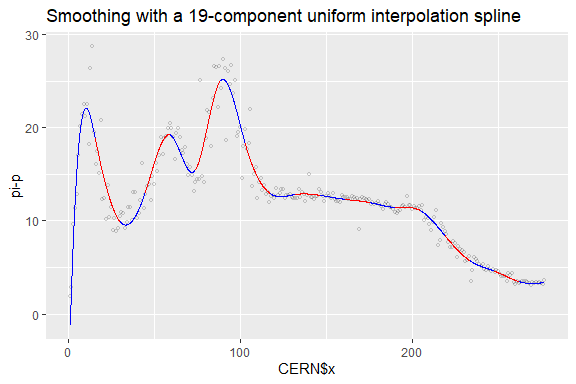It should be noted that the $$d$$ argument of the function can be used to control the direction of the first and last segments by defining the two exterior derivatives in advance.

We get e.g. the expression of the first spline segment as

sp$est_spline_polynomials ## List of polynomials: ## [] ## -7.773051 + 7.062941*x - 0.5219605*x^2 + 0.01142263*x^3 ### Specified external derivatives and forecasting with uniform interpolating splines Now we demonstrate how to forcast periodic data such as $$AirPassengers$$ using smoothing with interpolation splines of class $$C^2$$ and Hermite splines of class $$C^1$$. First we smooth the data using a 24-component uniform $$\textit{CICS}$$ yy <- as.vector( log10(AirPassengers) ) xx <- c(1:length(yy)) k <- 24 airp_spline <- cics_unif_explicit_smooth(xx, yy, k, c("blue","red") ,title=paste0("Smoothing log(AirPassengers) with a ", k,"-component uniform CICS"))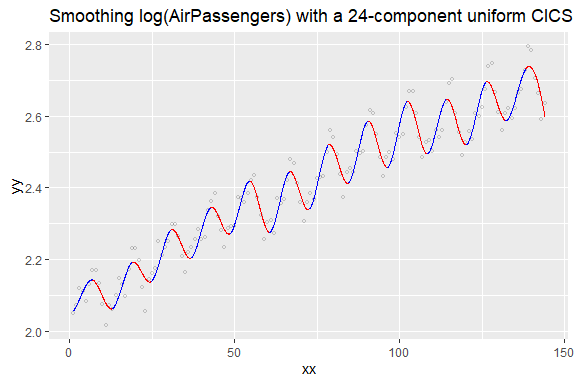from which the values and derivatives can be computed at any grid point. The function $$forecast\_demo$$ shows how to forcast the $$AirPassengers$$ data using a spline as $$airp\_spline$$ and an Hermite cubic spline ud <- forecast_demo()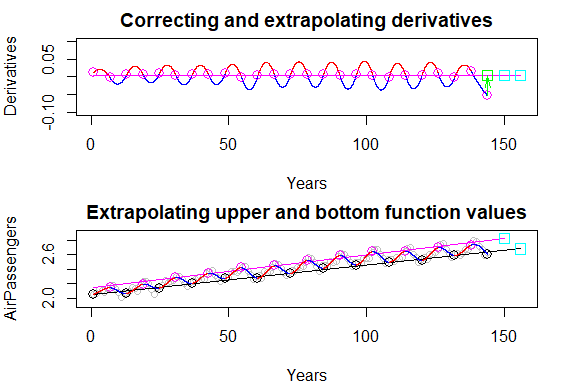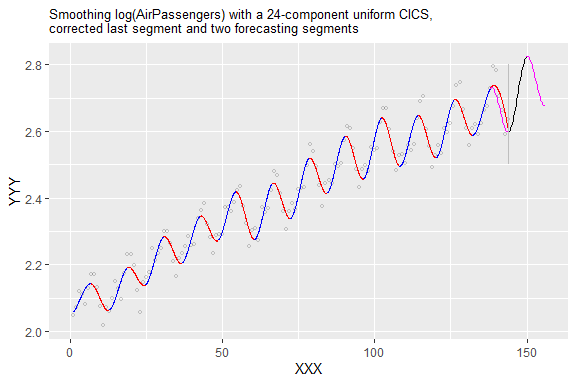The first two auxiliary plots depict the process of assessing three derivatives and two values for forecasting by an Hermite spline. All five values are denoted by boxes. As we see the first derivative of the last spline component decreases monotonously, so the derivative at the last gridpoint must be corrected. The first regression line was constructed from the derivatives of the smooting interpolation spline and the two others from the smooting interpolation spline at appropriate gridpoints. The above five values were computed using linear extrapolation. The last, third plot contains the prediction. • $$\mathbf{cics\_explicit\_smooth(xx, yy, uu, ...)}$$ Based on the uniform nodes of the uniform spline $$sp$$ sp <- cics_unif_explicit_smooth(CERN$x,CERN$y,19, #xlab = "x",ylab = "y", plotTF = FALSE); sp$nodes
##     1.00000  15.52632  30.05263  44.57895  59.10526  73.63158  88.15789
##   102.68421 117.21053 131.73684 146.26316 160.78947 175.31579 189.84211
##  204.36842 218.89474 233.42105 247.94737 262.47368 277.00000

one can easily propose (so far) by trial and error search an apropriate non-uniform grid with less nodes

uu <- c(1, 15, 26, 63, 73, 88, 103, 117,
132, 200, 203, 219, 258, 277)
sp <- cics_explicit_smooth(
xx = CERN$x, yy = CERN$y,
uu
#, d = c(5.57, 0.05)
)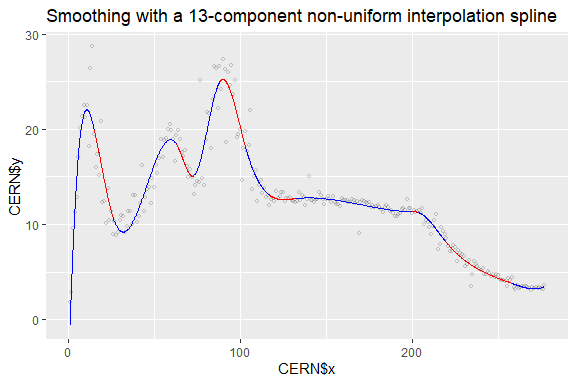## Supplementary functions - theoretical basics:

• $$\mathbf{tridiag\_inv\_general}$$

Below we provide three efficient ways to calculate the inversion of tridiagonal matrices. For computing the inverse of a general tridiagonal matrix in form \begin{align*} \mathbf{T}_n(\mathbf{a},\mathbf{b},\mathbf{c})=\begin{pmatrix} b_1 & c_1 & 0 &\dots & 0\\ a_1 & b_2 & c_1 & \dots & 0\\ 0 & a_2 & b_3 & \ddots & 0\\ 0 & 0 & \ddots & \ddots & c_{n-1}\\ 0 & 0 & \dots & a_{n-1} & b_n\\ \end{pmatrix}, \end{align*} the function $$\mathbf{tridiag\_inv\_general}$$ applyes Usmani’s theorem:

Let $$\mathbf{T}=\mathbf{T}_n(\mathbf{a},\mathbf{b},\mathbf{c})\in \mathbb{R}^{n\times n}$$, given by $$\eqref{tridiag}$$, be a regular tridiagonal matrix and let $$\mathbf{T}^{-1}=(\tau_{i,j})_{n\times n}$$. Then \begin{align*} \tau_{i,j}=\left\{ \begin{array}{lll} (-1)^{i+j}c_{i}c_{i+1}\dots c_{j-1}\frac{\theta_{i-1}\phi_{j+1}}{\theta_n}, & \quad i<j,\\ (-1)^{i+j}a_{j}a_{j+1}\dots a_{i-1}\frac{\theta_{j-1}\phi_{i+1}}{\theta_n}, & \quad i>j,\\ \frac{\theta_{i-1}\phi_{i+1}}{\theta_n}, & \quad i=j, \end{array} \right. \end{align*} where \begin{align*} \theta_0&=1,\ \theta_1=b_1,\ \theta_i=b_{i}\theta_{i-1}-a_{i-1}c_{i-1}\theta_{i-2},\ i=2,3,\dots,n;\\ \phi_{n+1}&=1,\ \phi_n=b_n,\ \phi_j=b_{j}\phi_{j+1}-a_{j}c_{j}\phi_{j+2},\ j=n-1,n-2,\dots,1. \end{align*}

$$~$$

• $$\mathbf{tridiag\_inv\_unif\_by\_sums}$$

For computing the inverse of a tridiagonal matrix of form $\mathbf{T}_{n}(a,b,a)=\begin{pmatrix} b & a & 0 &\dots & 0\\ a & b & a & \dots & 0\\ 0 & a & b & \ddots & 0\\ 0 & 0 & \ddots & \ddots & a\\ 0 & 0 & \dots & a & b\\ \end{pmatrix}$ the function $$\mathbf{tridiag\_inv\_unif\_by\_sums}$$ uses the theorem by Usmani (T1), combined with our own theorem (T2).

(T1) Let $$\mathbf{T}=\mathbf{T}_{n}(a,b,a)\in \mathbb{R}^{n\times n}$$ be a regular tridiagonal matrix, $$\mathbf{T}^{-1}=(\tau_{i,j})_{n\times n}$$. Then \begin{align} \tau_{i,j}=\left\{ \begin{array}{ll} (-1)^{i+j}a^{j-i}\frac{D_{i-1}D_{n-j}}{D_{n}}, & \quad i\leq j,\\ (-1)^{i+j}a^{i-j}\frac{D_{j-1}D_{n-i}}{D_{n}}, & \quad i>j,\\ \end{array} \right. \end{align} for $$D_{0}=1$$ and $$D_{k}=\mathrm{det}\mathbf{T}_{k}(a,b,c),\ k=1,2,\dots,n$$.

(T2) Let $$\mathbf{T}=\mathbf{T}_{n}(a,b,a)\in \mathbb{R}^{n\times n},\ n\geq 3,$$ be a regular matrix and let $$\mathbf{T}^{-1}=(\tau_{i,j}^{(n)})_{n\times n}$$. If $$i\leq j<n$$ and $$n-i\leq j$$, then \begin{align*} \tau_{i,j}^{(n)}=\tau_{i+1,j+1}^{(n)}+\tau_{i-n+j,n}^{(n)}. \end{align*}

$$~$$

• $$\mathbf{hermite\_bf\_matrix}$$

The function $$\mathbf{hermite\_bf\_matrix}$$ computes the one-component Hermite cubic spline, built for nodes $$u,v$$, function values $$y_u,y_v$$ and derivatives $$d_u,d_v$$, that is defined by: \begin{align*} s(x)&=y_{u}\frac{(x-v)^2}{(u-v)^2}\left(1-2\frac{x-u}{u-v}\right)+y_{v}\frac{(x-u)^2}{(v-u)^2}\left(1-2\frac{x-v}{v-u}\right)+\\ &+d_{u}\frac{(x-v)^2}{(u-v)^2}(x-u)+d_{v}\frac{(x-u)^2}{(v-u)^2}(x-v) \end{align*}

or by a matrix form: \begin{align*} s(x)=(1,x,x^2,x^3)\cdot \mathbf{H}(u,v)\cdot (y_u,y_{v},d_u,d_{v})^\mathsf{T}, \end{align*} where \begin{align*} \mathbf{H}(u,v)=\frac{1}{(v-u)^3}\begin{pmatrix} -3uv^2+v^3 & 3u^2v-u^3 & -uv^2(v-u) & -u^2v(v-u)\\ 6uv & -6uv & (2uv+v^2)(v-u) & (2uv+u^2)(v-u)\\ -3(u+v) & 3(u+v) & (-2v-u)(v-u) & (-2u-v)(v-u)\\ 2 & -2 & v-u & v-u\\ \end{pmatrix}. \end{align*}